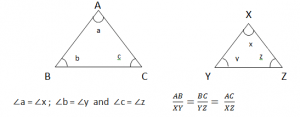Friday , November 22 2019
ONLINE BOOK STORE (Shop Now)Home / Igcse Mathematics Revision Notes / Congurence And Similarity

# Congurence And Similarity

### Congurency:Two geometric figures are congurent, if they are of the same shape and size.

AB =XY               ∠a = ∠x

BC = YZ               ∠b = ∠y

AC = XZ               ∠c = ∠z

### Tests of Congurency:

• Side Side Side (SSS) – all sides are equal
• Angle Angle Angle (AAA) – all angles are same
• Side Angle Side (SAS) – two sides and one angle are equal
• Right Angled – Hypotenuse – Side – one right angle and hyp & side equal

≡  – To denote “ is congruent to”

≢ – To denote “ is not congruent to”

### Similarity:

When two figures are similar, they are exactl the same in shape but not in size.

### Test:

• Equal corresponding Angles:

The angles of one triangle are equal to corresponding angles of the other triangle.• Proportional Corresponding Angles.
• Two Pairs of Proportional Corresponding Sides with equal angles.

We right similarity as ( ~ ) i.e ΔABC ~ ΔDEF

### Same Ratio:### Ratio of Volume: